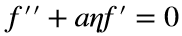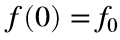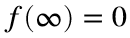# Problem 51322. Solve an ODE: diffusion problem 1

Problem
In the solution of a problem involving diffusion, the following ordinary differential equation ariseswhere a is a positive constant and primes denote differentiation with respect to the independent variable η. The problem has the conditionsand.
Write a function to solve this problem—that is, return values of f at specified values of η.
Background
The physical problem involves diffusion of a quantity from one point where the concentration is maintained at a constant value into a medium in which the concentration is zero far away. The ODE results from transforming the diffusion equation—a partial differential equation in time and a spatial coordinate—with a similarity solution.

### Solution Stats

31.58% Correct | 68.42% Incorrect
Last Solution submitted on Oct 04, 2023

### Community Treasure Hunt

Find the treasures in MATLAB Central and discover how the community can help you!

Start Hunting!